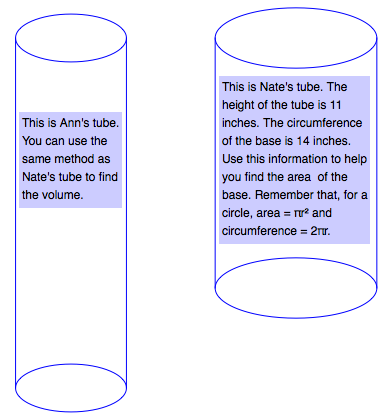### Home > MC1 > Chapter 10 > Lesson 10.2.3 > Problem10-100

10-100.

Ann and Nate's math class had a popcorn party. Each student made a container for popcorn by rolling up a piece of paper that was $11$ inches long by $14$ inches wide. Ann made a narrow tube by using the $14$-inch side for the height. Nate made his tube shorter and wider by using the $11$-inch side for the height.

Whose tube holds the most popcorn? How much more does it hold than the other tube?

This is Ann's tube. You can use the same method as Nate's tube to find the volume.

This is Nate's tube. The height of the tube is $11$ inches. The circumference of the base is $14$ inches. Use this information to help you find the area of the base. Remember that, for a circle, area $= πr^{2}$and circumference $= 2πr$.circumference $= 14 = 2πr$
radius $= 2.23$
area $= πr^{2} = π\left(2.23\right)^{2} = 15.60$
volume = (area of base) (height)
volume $= \left(15.60\right)\left(11\right) = 171.6$

Nate's tube has a volume of $171.6$ cubic inches and it holds more than Ann's.
Remember to show your calculations and to figure out how much more popcorn Nate's tube holds.